MATLAB信号处理——算法、仿真与实现2022-01

ISBN: 9787302579991

• MATLAB广泛用于数据分析、无线通信、深度学习、数据挖掘、图像处理、计算机视觉与信号处理等领 域。本书以数字信号处理为背景,系统讨论了 MATLAB在信号处理领域的知识与应用,具体包括数字信 号处理的基本理论、分析方法、算法的设计与实现、输出结果的数值与可视化展示等内容,涉及信号处理的 各个方面,是一本比较全面的参考书。 本书分为13章,全面系统地讨论了信号处理的相关问题。其中第1~7章介绍了信号的采集、Z变换、 离散傅里叶变换、快速傅里叶变换、噪声分布函数、IIR数字滤波器以及 FIR数字滤波器的相关知识和实现 方法;第8、9章着重对随机信号处理、功率谱分析、小波变换加以阐述;第10~13章介绍了 MATLAB在 信号处理领域主要工具箱的使用与设计过程。本书涉及的 MATLAB代码已经全部调试通过,所附结果均 为书中代码运行输出结果,并在代码中备注了较详尽的解释说明。 本书的特点是实用,知识内容与应用实例紧密结合,讲解深入浅出,实例程序既有单个函数的应用方 法,又包括整体系统的程序实现,同时也展示后的分析计算结果。本书可作为高校电子信息工程、通信 工程以及计算机科学与技术专业本科生和研究生的教学用书,也可作为科研技术人员的参考用书。 张轶  湖北沙市人，武汉纺织大学副教授，硕士生导师。多年来工作在教学与科研的线，主讲“MATLAB应用实践”“多媒体通信技术”“数据交换与传输”“无线传感网络”“计算机网络与仿真”“数据挖掘”“数学建模与仿真 ”“电磁场与电磁波”等本科生课程，以及“随机信号分析与仿真”“移动通信技术”等研究生课程。已发表教学研究类论文6篇，出版普通高等教育“十三五”规划教材2部，主持省级教学研究与改革项目2项，以及教育部高等学校电子信息专业教学指导委员会教学改革研究项目1项，主持完成中国纺织工业联合会教研项目1项、校级研究生教育教学研究项目1项，指导完成湖北省大学生创新创业实践项目1项，连续两年指导本科生毕业设计获得省级优秀学士论文。荣获院级青年教师讲课比赛二等奖，武汉纺织大学本科教学质量一等奖。 ●章 信号采样与波形实现

1.1 基本信号波形的MATLAB实现

1.2 信号的MATLAB运算处理

1.3 LTI连续系统信号时域分析与MATLAB实现

1.4 LTI离散系统信号时域分析与MATLAB实现

1.5 本章小结

第2章 Z变换与MATLAB信号处理

2.1 函数的Z变换与MATLAB处理

2.2 函数的逆Z变换与MATLAB处理

2.3 residuez函数的部分分式展开

2.4 系统零极点分析与MATLAB实现

2.5 离散系统频域响应分析与MATLAB实现

2.6 离散系统的差分解析与MATLAB实现

2.7 本章小结

第3章 离散傅里叶变换与MATLAB实现

3.1 有限长序列处理与MATLAB实现

3.2 N点离散傅里叶变换与MATLAB实现

3.3 序列圆周积分与MATLAB实现

3.4 本章小结

第4章 快速傅里叶变换与MATLAB实现

4.1 FFT函数调用格式

4.2 基于FFT的噪声信号分析与MATLAB实现

4.3 高斯脉冲信号分析与MATLAB实现

4.4 三角函数FFT分析与MATLAB实现

4.5 FFT快速卷积与MATLAB实现

4.6 本章小结

第5章 噪声信号分布函数与MATLAB实现

5.1 均匀分布

5.2 正态分布

5.3 卡方分布

5.4 F分布

5.5 T分布

5.6 指数分布

5.7 伽马分布

5.8 对数正态分布

5.9 瑞利分布

5.10 威布尔分布

5.11 二项分布

5.12 几何分布

5.13 泊松分布

5.14 本章小结

第6章 无限冲激响应滤波器与MATLAB实现

6.1 IIR数字滤波器结构

6.1.1 IIR数字滤波器直接型结构及MATLAB实现

6.1.2 IIR数字滤波器级联型结构及MATLAB实现

6.1.3 IIR数字滤波器并联型结构及MATLAB实现

6.2 IIR数字滤波器基础——模拟低通滤波器结构及MATLAB实现

6.2.1 巴特沃斯滤波器结构及MATLAB实现

6.2.2 切比雪夫滤波器结构及MATLAB实现

6.2.3 IIR数字低通滤波器的MATLAB实现(基于脉冲响应不变法)

6.2.4 IIR数字低通滤波器的MATLAB实现(基于双线性变换法)

6.3 模拟低通滤波器的频率变换(高通、带通、带阻滤波器的变换)

本章小结

第7章 有限冲激响应滤波器与MATLAB实现

7.1 FIR数字滤波器结构

7.2 FIR数字滤波器窗函数设计及MATLAB实现

7.2.1 矩形窗MATLAB设计

7.2.2 三角窗MATLAB设计

7.2.3 汉宁窗MATLAB设计

7.2.4 汉明窗MATLAB设计

7.2.5 布莱克曼窗MATLAB设计

7.2.6 凯撒窗MATLAB设计

7.2.7 巴特窗MATLAB设计

7.2.8 滤波器窗函数设计比较

7.3 基于频率采样的FIR数字滤波器设计及MATLAB实现

7.4 其他类型的FIR数字滤波器设计及MATLAB实现

7.4.1 等纹波滤波器MATLAB设计

7.4.2 梳状滤波器MATLAB设计

7.4.3 维纳滤波器MATLAB设计

7.4.4 Kalman(卡尔曼)滤波器MATLAB设计

7.4.5 自适应滤波器MATLAB设计

7.4.6 Lattice滤波器MATLAB设计

7.5 本章小结

第8章 随机信号处理与MATLAB实现

8.1 随机信号处理MATLAB基本函数

8.2 随机信号功率谱分析与MATLAB实现

8.2.1 经典谱估计

8.2.2 参数谱估计

8.2.3 AR谱估计

8.3 本章小结

第9章 基于小波分析的信号处理与MATLAB实现

9.1 小波变换的MATLAB实现

9.2 小波信号分析

9.3 基于提升方案的MATLAB信号分析

9.3.1 一维提升小波分解及重构lwt&ilwt

9.3.2 二维提升小波分解及重构lwt2&ilwt2

9.3.3 提升或重构小波系数lwtcoef&lwtcoef2

9.4 小波去噪实现及MATLAB应用

9.4.1 基于小波变换的一维信号降噪

9.4.2 降噪压缩的小波变换默认阈值获取

9.4.3 一维或二维信号全局阈值降噪压缩

9.5 小波变换与傅里叶变换比较

9.6 小波工具箱应用

9.6.1 基于小波工具箱的一维小波包分析

9.6.2 基于小波工具箱的Wavelet 1-D分析

9.7 本章小结

第10章 语音信号处理与MATLAB实现

10.1 语音信号模型

10.2 语音信号的特点与采集

10.3 短时傅里叶变换的频谱分析

10.4 语音信号压缩与去噪处理

10.5 本章小结

第11章 数字图像信号去噪处理与MATLAB实现

11.1 图像导入的基本MATLAB函数

11.2 图像增强及MATLAB分析

11.3 本章小结

第12章 场论信号的MATLAB处理

12.1 标量场的MATLAB可视化

12.2 矢量场的MATLAB可视化

12.3 梯度、散度、旋度的MATLAB可视化

12.4 拉普拉斯运算的MATLAB可视化

12.5 基于PDEtool求解微分方程的MATLAB可视化

12.6 本章小结

13.4 本章小结

参考文献

• 内容简介:
MATLAB广泛用于数据分析、无线通信、深度学习、数据挖掘、图像处理、计算机视觉与信号处理等领 域。本书以数字信号处理为背景,系统讨论了 MATLAB在信号处理领域的知识与应用,具体包括数字信 号处理的基本理论、分析方法、算法的设计与实现、输出结果的数值与可视化展示等内容,涉及信号处理的 各个方面,是一本比较全面的参考书。 本书分为13章,全面系统地讨论了信号处理的相关问题。其中第1~7章介绍了信号的采集、Z变换、 离散傅里叶变换、快速傅里叶变换、噪声分布函数、IIR数字滤波器以及 FIR数字滤波器的相关知识和实现 方法;第8、9章着重对随机信号处理、功率谱分析、小波变换加以阐述;第10~13章介绍了 MATLAB在 信号处理领域主要工具箱的使用与设计过程。本书涉及的 MATLAB代码已经全部调试通过,所附结果均 为书中代码运行输出结果,并在代码中备注了较详尽的解释说明。 本书的特点是实用,知识内容与应用实例紧密结合,讲解深入浅出,实例程序既有单个函数的应用方 法,又包括整体系统的程序实现,同时也展示后的分析计算结果。本书可作为高校电子信息工程、通信 工程以及计算机科学与技术专业本科生和研究生的教学用书,也可作为科研技术人员的参考用书。
• 作者简介:
张轶  湖北沙市人，武汉纺织大学副教授，硕士生导师。多年来工作在教学与科研的线，主讲“MATLAB应用实践”“多媒体通信技术”“数据交换与传输”“无线传感网络”“计算机网络与仿真”“数据挖掘”“数学建模与仿真 ”“电磁场与电磁波”等本科生课程，以及“随机信号分析与仿真”“移动通信技术”等研究生课程。已发表教学研究类论文6篇，出版普通高等教育“十三五”规划教材2部，主持省级教学研究与改革项目2项，以及教育部高等学校电子信息专业教学指导委员会教学改革研究项目1项，主持完成中国纺织工业联合会教研项目1项、校级研究生教育教学研究项目1项，指导完成湖北省大学生创新创业实践项目1项，连续两年指导本科生毕业设计获得省级优秀学士论文。荣获院级青年教师讲课比赛二等奖，武汉纺织大学本科教学质量一等奖。
• 目录:
●章 信号采样与波形实现

1.1 基本信号波形的MATLAB实现

1.2 信号的MATLAB运算处理

1.3 LTI连续系统信号时域分析与MATLAB实现

1.4 LTI离散系统信号时域分析与MATLAB实现

1.5 本章小结

第2章 Z变换与MATLAB信号处理

2.1 函数的Z变换与MATLAB处理

2.2 函数的逆Z变换与MATLAB处理

2.3 residuez函数的部分分式展开

2.4 系统零极点分析与MATLAB实现

2.5 离散系统频域响应分析与MATLAB实现

2.6 离散系统的差分解析与MATLAB实现

2.7 本章小结

第3章 离散傅里叶变换与MATLAB实现

3.1 有限长序列处理与MATLAB实现

3.2 N点离散傅里叶变换与MATLAB实现

3.3 序列圆周积分与MATLAB实现

3.4 本章小结

第4章 快速傅里叶变换与MATLAB实现

4.1 FFT函数调用格式

4.2 基于FFT的噪声信号分析与MATLAB实现

4.3 高斯脉冲信号分析与MATLAB实现

4.4 三角函数FFT分析与MATLAB实现

4.5 FFT快速卷积与MATLAB实现

4.6 本章小结

第5章 噪声信号分布函数与MATLAB实现

5.1 均匀分布

5.2 正态分布

5.3 卡方分布

5.4 F分布

5.5 T分布

5.6 指数分布

5.7 伽马分布

5.8 对数正态分布

5.9 瑞利分布

5.10 威布尔分布

5.11 二项分布

5.12 几何分布

5.13 泊松分布

5.14 本章小结

第6章 无限冲激响应滤波器与MATLAB实现

6.1 IIR数字滤波器结构

6.1.1 IIR数字滤波器直接型结构及MATLAB实现

6.1.2 IIR数字滤波器级联型结构及MATLAB实现

6.1.3 IIR数字滤波器并联型结构及MATLAB实现

6.2 IIR数字滤波器基础——模拟低通滤波器结构及MATLAB实现

6.2.1 巴特沃斯滤波器结构及MATLAB实现

6.2.2 切比雪夫滤波器结构及MATLAB实现

6.2.3 IIR数字低通滤波器的MATLAB实现(基于脉冲响应不变法)

6.2.4 IIR数字低通滤波器的MATLAB实现(基于双线性变换法)

6.3 模拟低通滤波器的频率变换(高通、带通、带阻滤波器的变换)

本章小结

第7章 有限冲激响应滤波器与MATLAB实现

7.1 FIR数字滤波器结构

7.2 FIR数字滤波器窗函数设计及MATLAB实现

7.2.1 矩形窗MATLAB设计

7.2.2 三角窗MATLAB设计

7.2.3 汉宁窗MATLAB设计

7.2.4 汉明窗MATLAB设计

7.2.5 布莱克曼窗MATLAB设计

7.2.6 凯撒窗MATLAB设计

7.2.7 巴特窗MATLAB设计

7.2.8 滤波器窗函数设计比较

7.3 基于频率采样的FIR数字滤波器设计及MATLAB实现

7.4 其他类型的FIR数字滤波器设计及MATLAB实现

7.4.1 等纹波滤波器MATLAB设计

7.4.2 梳状滤波器MATLAB设计

7.4.3 维纳滤波器MATLAB设计

7.4.4 Kalman(卡尔曼)滤波器MATLAB设计

7.4.5 自适应滤波器MATLAB设计

7.4.6 Lattice滤波器MATLAB设计

7.5 本章小结

第8章 随机信号处理与MATLAB实现

8.1 随机信号处理MATLAB基本函数

8.2 随机信号功率谱分析与MATLAB实现

8.2.1 经典谱估计

8.2.2 参数谱估计

8.2.3 AR谱估计

8.3 本章小结

第9章 基于小波分析的信号处理与MATLAB实现

9.1 小波变换的MATLAB实现

9.2 小波信号分析

9.3 基于提升方案的MATLAB信号分析

9.3.1 一维提升小波分解及重构lwt&ilwt

9.3.2 二维提升小波分解及重构lwt2&ilwt2

9.3.3 提升或重构小波系数lwtcoef&lwtcoef2

9.4 小波去噪实现及MATLAB应用

9.4.1 基于小波变换的一维信号降噪

9.4.2 降噪压缩的小波变换默认阈值获取

9.4.3 一维或二维信号全局阈值降噪压缩

9.5 小波变换与傅里叶变换比较

9.6 小波工具箱应用

9.6.1 基于小波工具箱的一维小波包分析

9.6.2 基于小波工具箱的Wavelet 1-D分析

9.7 本章小结

第10章 语音信号处理与MATLAB实现

10.1 语音信号模型

10.2 语音信号的特点与采集

10.3 短时傅里叶变换的频谱分析

10.4 语音信号压缩与去噪处理

10.5 本章小结

第11章 数字图像信号去噪处理与MATLAB实现

11.1 图像导入的基本MATLAB函数

11.2 图像增强及MATLAB分析

11.3 本章小结

第12章 场论信号的MATLAB处理

12.1 标量场的MATLAB可视化

12.2 矢量场的MATLAB可视化

12.3 梯度、散度、旋度的MATLAB可视化

12.4 拉普拉斯运算的MATLAB可视化

12.5 基于PDEtool求解微分方程的MATLAB可视化

12.6 本章小结

13.4 本章小结

参考文献MATLAB程序设计及应用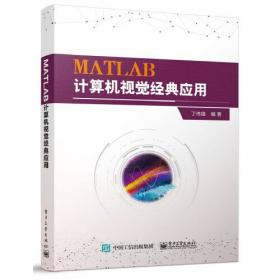MATLAB计算机视觉经典应用MATLAB图像处理与应用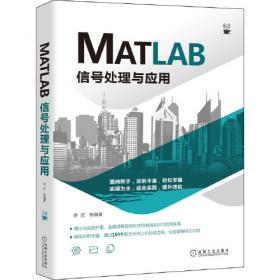MATLAB信号处理与应用MATLAB函数及应用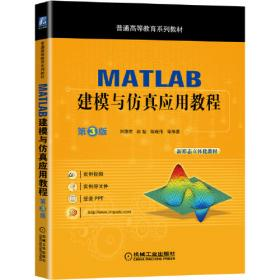MATLAB建模与仿真应用教程 第3版MARUGOTO日本的语言与文化(初级1)(A2)(理解篇)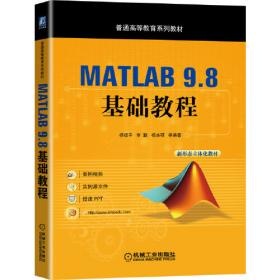MATLAB 9.8 基础教程MARUGOTO日本的语言与文化(初级1)(A2)(活动篇)MATLAB 2020 数学计算从入门到精通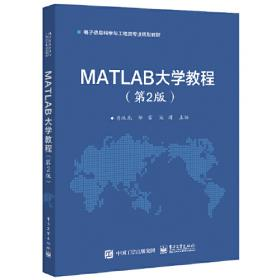MATLAB大学教程（第2版）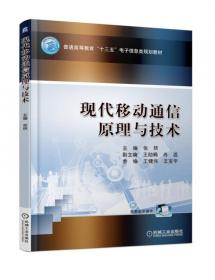1990